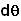## Wednesday, June 11, 2008

### Irodov Solution 1.181The first thing to realize is that since the mass is always ejected perpendicular tot the direction of motion of the rocket, the ejection will only change the direction of motion of the rocket and not its magnitude of tangential velocity (velocity along its direction of motion). In other words the speed of the rocket will never change and always remain v0. This is not very different from a stone being rotated by a string where the tension in the string always acts perpendicular to the motion of the stone and so only changes its direction.

Suppose that an infinitesimally small mass is ejected out of the rocket and as a result say the rocket acquires a velocity dv in the perpendicular direction. The equation relating dm and dv is given by equation in 1.179 i.e.The negative sign comes because, in the derivation in 1.179 we assumed that u and dv are in the same direction. In this problem, as shown in the figure, u and dv are in opposite directions. Now if the rocket acquires a velocity dv in the perpendicular direction, as shown in the figure, the rocket changes its direction of motion by an anglegiven by,From (2) and (3) we have,